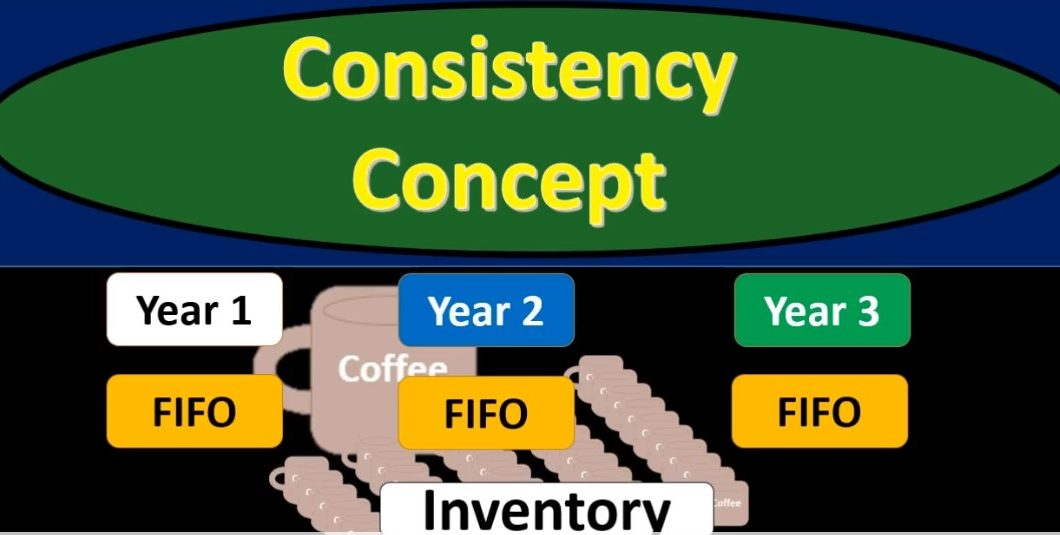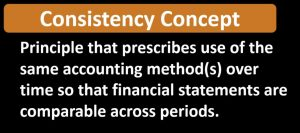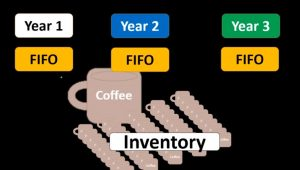## Consistency Concept

In this presentation we will discuss the consistency principle as it relates to inventory and inventory assumptions. First, we’re going to define the consistency principle and then apply it to an assumption such as the flow assumption such as do we use something like a first out last In First Out average inventory system, the definition of consistency principle according to fundamental accounting principles, while 22nd edition is a principle that prescribes use of the same accounting method methods over time so that financial statements are comparable across periods. So, here we’re considering the assumptions that we’re making with the flow of inventory those being either first in first out last in first out or the average method typically for the cost flow assumptions, because those are assumptions.

00:56

They do have an effect on the financial statements and therefore We want to make sure that we are consistent in those meaning that if we used something such as in year one, a first in first out inventory flow assumption in year two, ideally we would want the same assumption, as well as year three and so forth. However, if we we do have an incentives, companies often have an incentive possibly to shift assumptions such as the inventory flow assumption, to do things like increase or decrease their net income in a particular timeframe. We can see that if we change the first in first out assumption, remember that as we look at the first in first out assumption as compared to the lastin first out assumption, that if there were a period of rising prices, the net income would be higher under a first in first out assumption and lower for lastin.01:54

First out assumption, and therefore if we were to switch back and forth between flow assumption like these, we could manipulate that net income in some ways. for whatever purpose we would like either companies may have an incentive to try to increase net income to look better on the financial statements, or sometimes decrease that net income in order to look worse possibly for lowering tax purposes. And of course, from a reading the financial statement purpose, from analyzing the financial statements from being from the perspective of being able to prepare financial statements from period to period from year to year, then we want consistency. We want those those cost flow assumptions to be the same across time. So that’s going to be the consistency principle.02:40

Now, could a company ever change their flow assumption? If the flow assumption is thought to be better if there’s some reason to change the flow assumption that is not just to adjust or have the income be different during a time period but in other words, For better reporting purposes, then that would be a more legitimate reason to make a one time change of a cost flow assumption. But what we don’t want to see clearly would be cost flow assumptions changing consistently over time, in order to change basically, the flow assumptions and the effect on the financial statements particularly the timing statements on the income statement and the net income due to the effect in cost of goods sold. Therefore, once we have a cost flow assumption, we expect to and want to stay with that cost flow assumptions so that we have the ability to compare year to year month to month time period to time period.